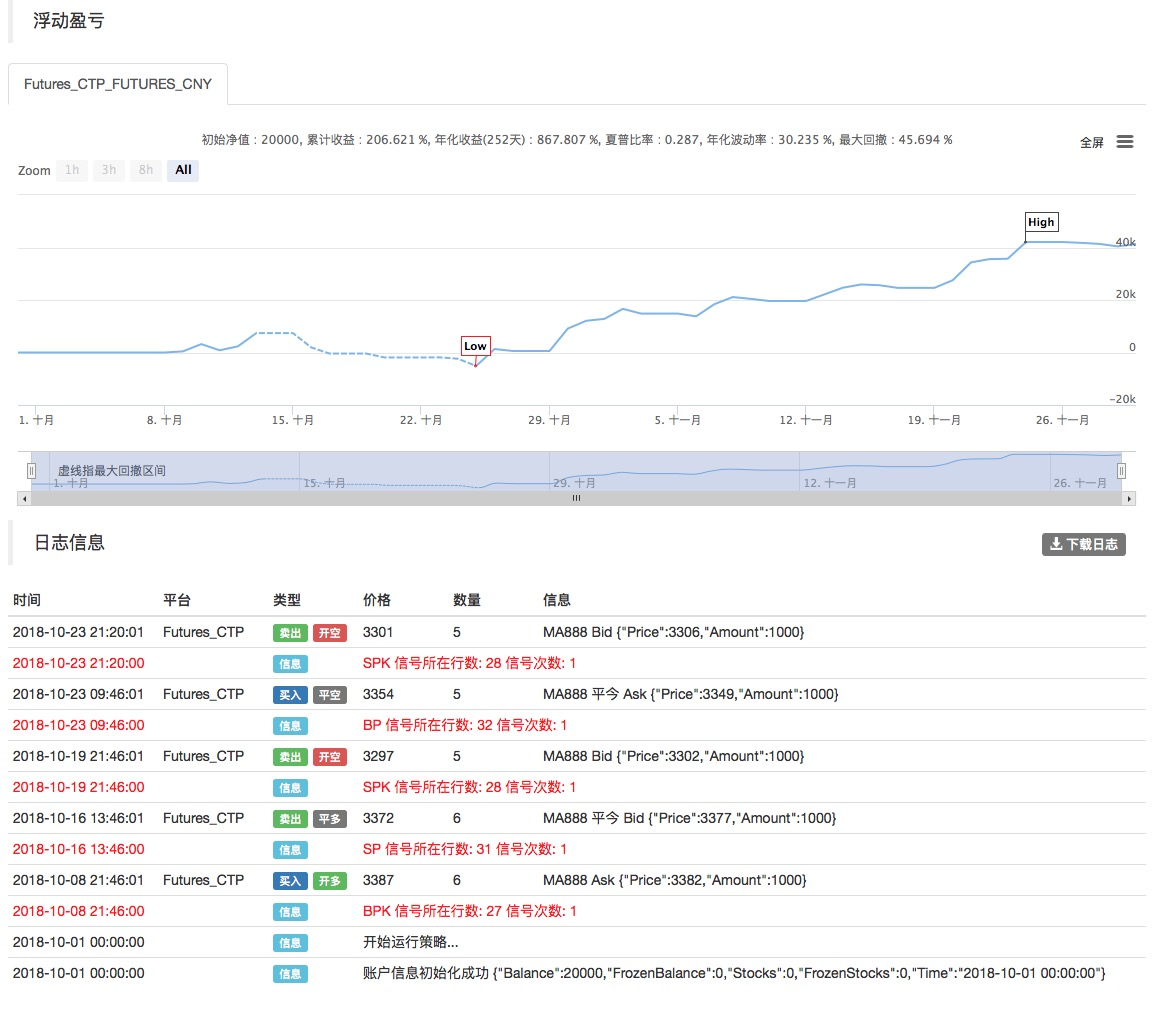# 均幅指标策略框架

Author: , Created: 2019-07-19 10:26:37, Updated: 2019-07-20 21:22:41t——当日； n——时间长度； Ci——第i日的收盘价； Hi——第i日的最高价； Li——第i日的最低价。

``````LOTS:=MAX(1,INTPART(MONEYTOT/(O*UNIT*0.1)));
C_O:EMA(C,N)-EMA(O,N);
B:=CROSSUP(C_O,0);
S:=CROSSDOWN(C_O,0);
TR:=MAX(MAX((H-L),ABS(REF(C,1)-H)),ABS(REF(C,1)-L));
ATR:MA(TR,N);
BAND:=ATR*0.1*M;
PRICE_BPK:=VALUEWHEN(B,H+BAND);
PRICE_SP:=VALUEWHEN(B,L-BAND);
PRICE_SPK:=VALUEWHEN(S,L-BAND);
PRICE_BP:=VALUEWHEN(S,H+BAND);

// 策略逻辑
// strategy logic
BARPOS>N AND C_O>0  AND C>=PRICE_BPK,BPK(LOTS);
BARPOS>N AND C_O<0  AND C<=PRICE_SPK,SPK(LOTS);

// 下单
// place an order
S,SP(BKVOL);
B,BP(SKVOL);

C<=PRICE_SP,SP(BKVOL);
C>=PRICE_BP,BP(SKVOL);
``````More